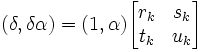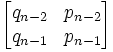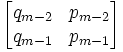## Wednesday, January 04, 2006

### Continued Fractions and Matrices: More Lemmas

Today's blog continues exploring properties of continued fractions and matrices which are used in the solution for Pell's Equation. This blog assumes that you have already read the previous blog.

The details of today's blog is based on Harold M. Stark's An Introduction to Number Theory.

Lemma 1: if δ(1,√d) = (1,√d)Mn-1Mn+kj, then there exists rk, sk, tk, uk such that:and

(dtk - sk) + (rk - uk)√d = 0.

(1) By assumption, we let:
δ(1,√d) = (1,√d)Mn-1Mn+kj [See here for an example where this assumption is true]

(2) Further, since we know that Mn-1 is a 2 x 2 matrix (see here), we know that the product with Mn+kj is a 2x2 matrix (see here) and we can assume that there exists rk, sk, tk, uk such that:(3) Now, we know that:
δ(1,α) = (δ,δα) [See here for review of matrix math]

(4) From a previous result, we know that:
δ(1,α) = (1,α)Mn-1Mn+j [See here]

(5) Combining #4 and #5 with #2 gives us:(6) From #6 (see here for review of matrix math), we can conclude that:

(δ, δα) = (rk+tkα, sk+ukα)

(7) This means that:

δ = rk + tkα

δα = sk + ukα

(8) Combining these two equations gives:

(rk + tkα)α = sk + ukα

(9) Which means that:

rkα + tkα2 = sk + ukα

(10) And subtracting the left-side value from both sides give us:

tkα2 + rkα - ukα - sk = 0

which is:

tkα2 + α(rk - uk) - sk = 0

(11) Since we know that α = √d (see here for review of α)

tkα2 + α(rk - uk) - sk = tkd +√d (rk - uk) - sk

(12) So, this gives us finally:
(dtk - sk) + (rk - uk)√d= 0.

QED

Lemma 2: Mn = Mm → n = m.

(1) Assume that Mn = Mm but n ≠ m

(2) Mn =(3) Mm =(4) Since Mn = Mm, we have:

qn-2 = qm-2
qn-1 = qm-1

(5) Now q1 is less than q2 and so on (see here for proof). So, m-2, n-2, n-1, m-1 must all be less than 1. (Otherwise, they cannot be equal)

(6) q0 and q-2 = 1 while q-1 = 0.

(7) So, m-2, n-2, n-1, m-1 cannot equal -1. [Since by #6 no other value = 0]

(8) On the other, it is possible for q-2 = q0 = q1 since all could = 1. (See here and here)

(9) So n-2, m-2, n-1, m-1 must equal -2,0, or 1.

(10) So n must = 2 (so that n-1 = 1, n-2 = 0). [Note: n=0 doesn't work since n-1 = -1; n=1 doesn't work since n-2=-1; and n=3 doesn't work since n-1 = 2]

(11) But then m must also = 2 (so that m-1=1, m-2=0) but this goes against our assumption.

(12) So we have a contradiction. There is no way that Mn = Mm without n = m.

QED

#### 1 comment:

Scouse Rob said...

Step (6) of Lemma 1 references itself instead of step (5).

Rob